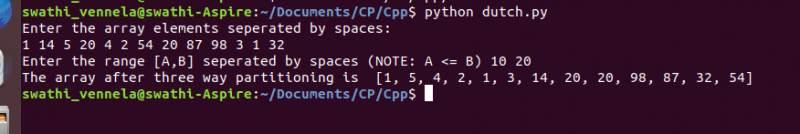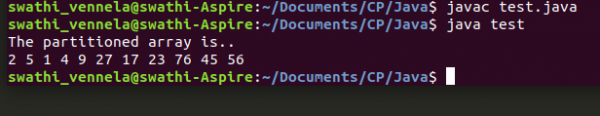In this tutorial, we will see how to do three way partitioning of an array around a given range.

## What is Three way partitioning?

Given an array, and a range, say, `[A,B]`, the task is to partition the array into three parts such that,

• All the elements of the array that are less than A appear in the first partition
• All the elements of the array that lie in the range of A to B appear in the second partition, and,
• All the elements of the array that are greater than B come in the third partition

Note that, `A<=B` and, the individual elements in each of the partitions needn’t necessarily be sorted.

Does this sound similar to quick sort?

Yes, it is. This is very similar to the partition step of quick sort. In quick sort, we pick a pivot element in an array, and place the pivot in the right index such that, all the elements lesser than the pivot come to its left and all the elements greater than the pivot come to its right. This can be compared to three-way partitioning, where pivot is not just an element, but it is a range `[A,B]`.

Now, let us see how this problem can be done..

## Naive Approach

This can be solved by sorting the array. But, that will take order of `nlogn` time. (where n is the size of the array). Can we think of a more efficient solution in a single pass without actually sorting the array?

## Efficient Approach

• Take three pointers `low`, `mid` and `high`.
• Set `low and mid` to the 0th index, and `high` to the `(n-1)th` index initially.
• Say, the partition containing all the elements less than A is the first partition and the block containing all the elements greater than B is the third partition.
• Use “low” to maintain the boundary of the first partition and “high” to maintain the boundary of the third partition.
• While, “mid” iterates over the array and swaps the right elements to the first and third partition.
• The resulting array is divided into 3 partitions.

## Implementation

• Initialize `low=0`, `mid=0` and `high=n-1`.
• Use mid to iterate over the array and visit each element. At an element `arr[mid]`,
• `if arr[mid]` is less than A, then, swap it with `arr[low]` and increment both low and mid by one.
• `if arr[mid]` is greater than B, swap it with `arr[high]` and decrement high by one.
• Otherwise, increment `mid` by one.
• The resulting array is the partitioned array.

## Example:

``````def threeWayPartition(array, a, b):
low=0
mid=0
high=len(array)-1

while(mid <= high):
if(array[mid] < a): #swap array[mid] with array[low]
temp = array[mid]
array[mid] = array[low]
array[low] = temp
low += 1
mid += 1
elif(array[mid] > b):
#swap array[mid] with array[high]
temp = array[mid]
array[mid] = array[high]
array[high] = temp
high -= 1
else:
mid += 1
return array

if __name__ == "__main__":
print("Enter the array elements seperated by spaces: ")
str_arr = input().split(' ')
arr = [int(num) for num in str_arr]
a, b = [int(x) for x in input("Enter the range [A,B] seperated by spaces (NOTE: A <= B) ").split()]
arr = threeWayPartition(arr, a, b)
print("The array after three way partitioning is ", arr)``````

### Output``````import java.util.*;
import java.io.*;

public class test{
public static void main(String[] args){
int arr[] = {2,5,27,56,17,4,9,23,76,1,45};
int n = arr.length, a=15, b=30;
int low=0, mid=0, high=n-1, temp;

while(mid<=high){
if(arr[mid] < a){
//swap arr[mid] and arr[low]
temp = arr[mid];
arr[mid] = arr[low];
arr[low] = temp;
low++;
mid++;
} else if(arr[mid] > b){
//swap arr[mid] and arr[high]
temp = arr[mid];
arr[mid] = arr[high];
arr[high] = temp;
high--;
} else
mid++;
}
System.out.println("The partitioned array is..");
for(int i=0;i<arr.length; i++)
System.out.print(arr[i]+" ");
System.out.println();
}
}``````

### Output## Time and space complexity

The time complexity of this algorithm is in the order of n, i.e., `O(n)` as it is a single pass algorithm. The algorithm is in-place and doesn’t take any extra space, making the space complexity constant i.e., `O(1)`.

## Applications

One of the important applications of this algorithm is, to sort an array with three types of elements where n>=3. Say, we have an array of 0s, 1s and 2s. Then, this approach can be employed to sort it in a single pass with constant space complexity. In this case, A=1 and B=1.

## References:

Happy Learning 🙂